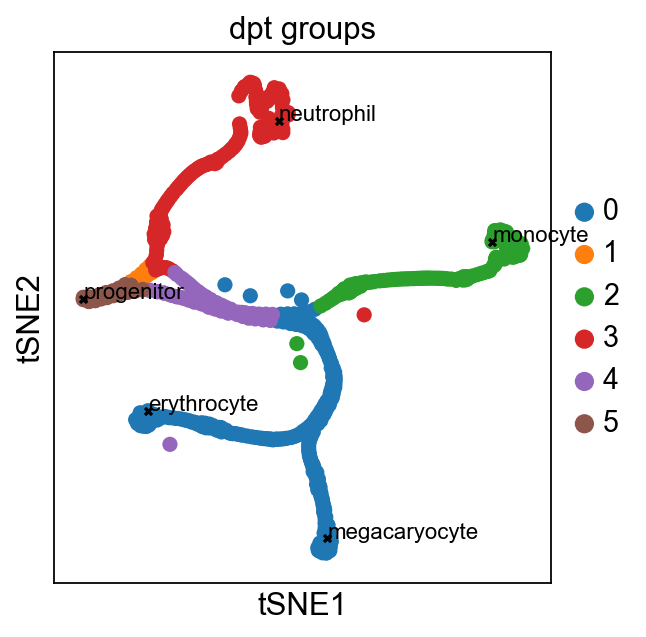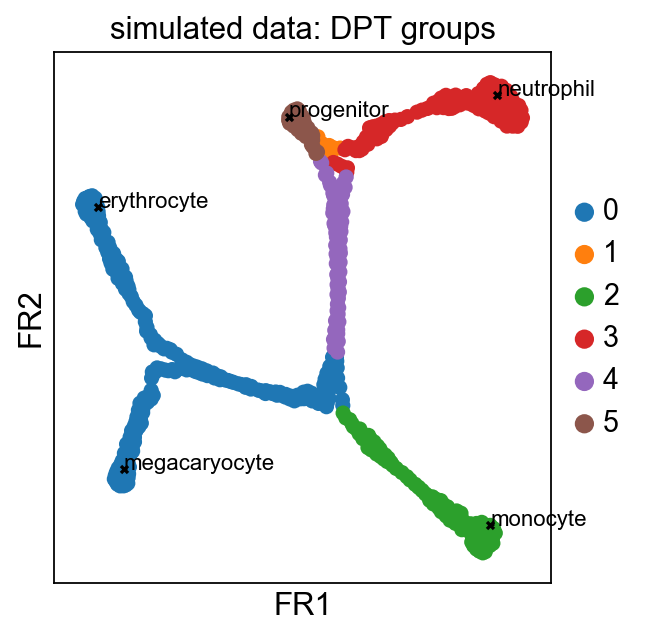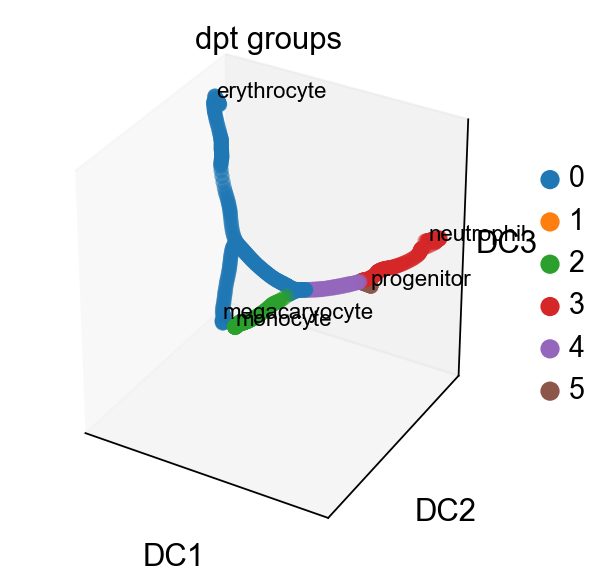First compiled on: December 3, 2016.

# Simulating myeloid progenitors¶

In :
import numpy as np
import scanpy.api as sc

sc.settings.verbosity = 3  # verbosity: errors (0), warnings (1), info (2), hints (3)
sc.settings.set_figure_params(dpi=80)  # low dpi (dots per inch) yields small inline figures
sc.logging.print_versions()

scanpy==1.0 anndata==0.5.8 numpy==1.14.1 scipy==1.0.0 pandas==0.22.0 scikit-learn==0.19.1 statsmodels==0.8.0 python-igraph==0.7.1 louvain==0.6.1


Here, we simulate data using a literature-curated boolean gene regulatory network, which is believed to describe myeloid differentiation (Krumsiek et al., 2011). Using sim, the boolean model

Gata2 = Gata2 and not (Gata1 and Fog1) and not Pu.1
Gata1 = (Gata1 or Gata2 or Fli1) and not Pu.1
Fog1 = Gata1
EKLF = Gata1 and not Fli1
Fli1 = Gata1 and not EKLF
SCL = Gata1 and not Pu.1
Cebpa = Cebpa and not (Gata1 and Fog1 and SCL)
Pu.1 = (Cebpa or Pu.1) and not (Gata1 or Gata2)
cJun = Pu.1 and not Gfi1
EgrNab = (Pu.1 and cJun) and not Gfi1
Gfi1 = Cebpa and not EgrNab

is translated into a stochastic differential equation (Wittmann et al., 2009). Simulations result in branching time series of gene expression, where each branch corresponds to a certain cell fate of common myeloid progenitors (megakaryocytes, erythrocytes, granulocytes and monocytes).

Instead of simulating the data as in the next line, you can also retrieve it from the builtin examples

adata = sc.datasets.krumsiek11()

This uses the default parameters from here. To change parameters, read in a different parameter file or simply pass them to sc.tl.sim.

In :
adata = sc.tl.sim('krumsiek11')

reading params file /Users/alexwolf/_hholtz/01_projects/1512_scanpy/scanpy/scanpy/sim_models/krumsiek11_params.txt
Observation names are not unique. To make them unique, call .obs_names_make_unique.


Plot the four realizations of time series.

In :
sc.pl.sim(adata)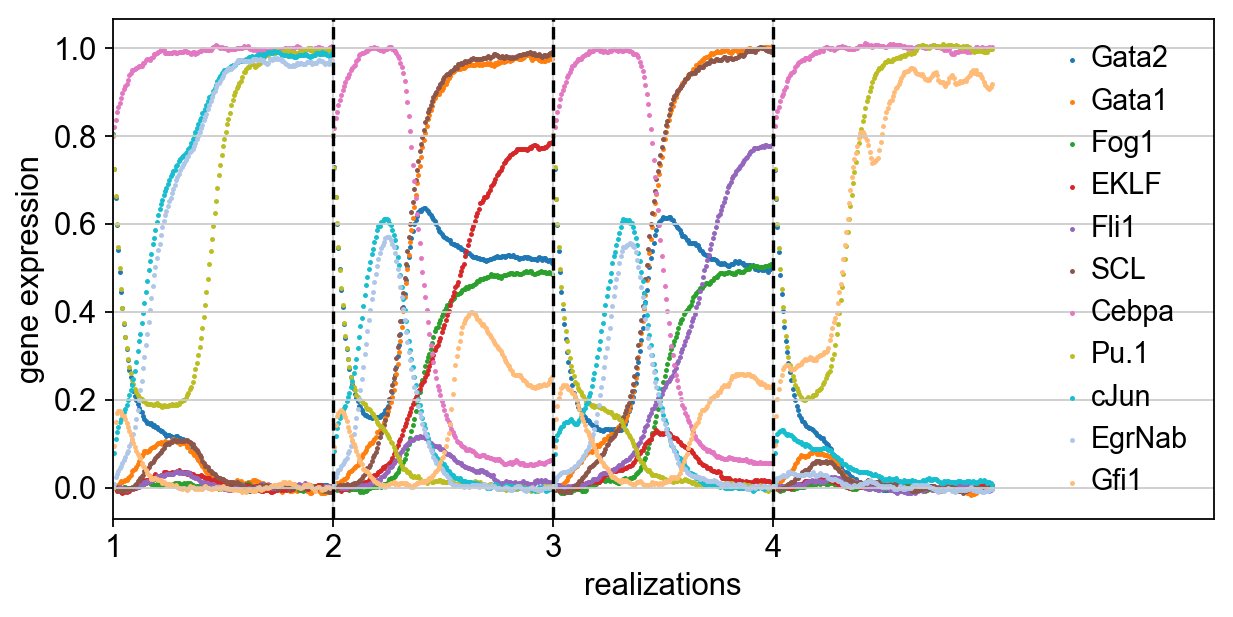Compute further visualizations.

In :
sc.tl.tsne(adata)

computing tSNE
using data matrix X directly
using the 'MulticoreTSNE' package by Ulyanov (2017)
computing neighbors
using data matrix X directly
finished (0:00:02.652) --> added to .uns['neighbors']
drawing single-cell graph using layout "fr"

In :
sc.pp.neighbors(adata, n_neighbors=30)

computing neighbors
initialized .distances .connectivities
using data matrix X directly
finished (0:00:00.303) --> added to .uns['neighbors']
drawing single-cell graph using layout "fr"


Inspecting the genes of the fixed poitns, we can make the following annotation.

In :
fate_labels = {0: 'progenitor', 159: 'monocyte', 319: 'erythrocyte',
459: 'megacaryocyte', 619: 'neutrophil'}
cell_type = np.array(['progenitor' for i in range(adata.n_obs)])
cell_type[80:160] = 'monocyte'
cell_type[240:320] = 'erythrocyte'
cell_type[400:480] = 'megakaryocyte'
cell_type[560:640] = 'neutrophil'

In :
sc.pl.tsne(adata)

... storing 'cell_type' as categorical type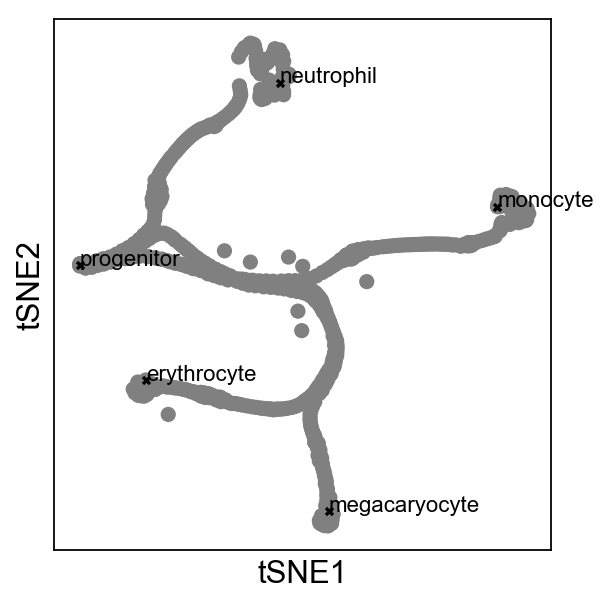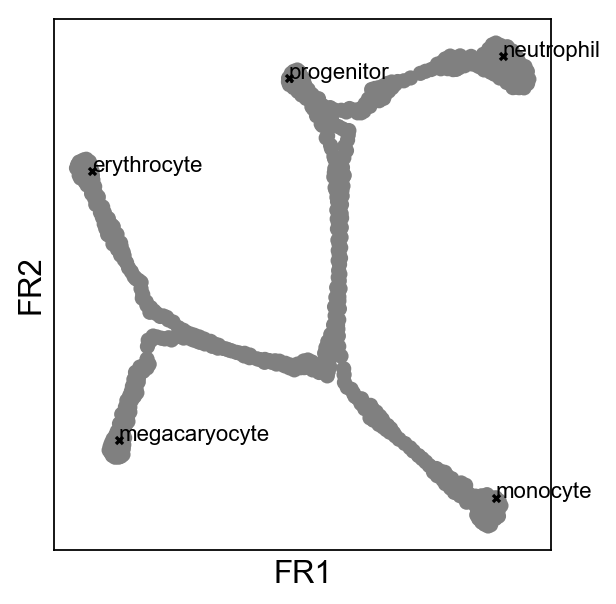In :
sc.pl.tsne(adata, color='cell_type')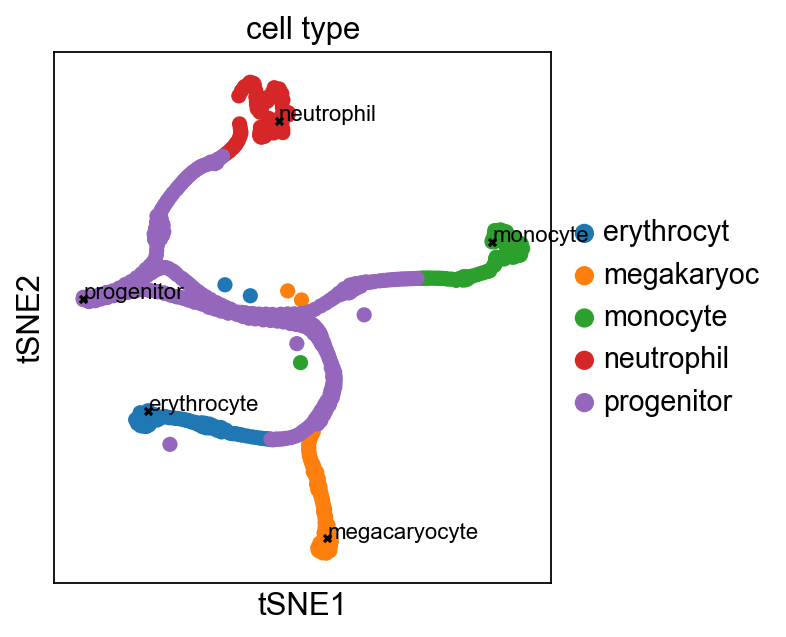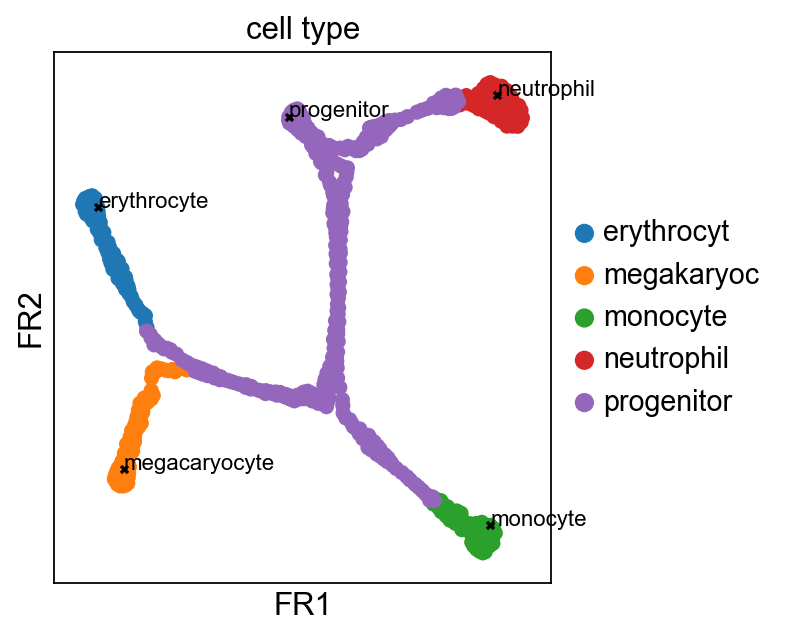### Reconstructing progression and branching using DPT¶

In :
adata.uns['iroot'] = 0

performing Diffusion Pseudotime analysis
initialized .distances .connectivities
eigenvalues of transition matrix
[ 1.            0.9994561076  0.9990158081  0.9974680543  0.9971337318
0.9936081171  0.9897011518  0.983776629   0.9828016162  0.9784392118]
this uses a hierarchical implementation
'dpt_groups', the branching subgroups of dpt (adata.obs)

In :
sc.pl.tsne(adata, color='dpt_pseudotime')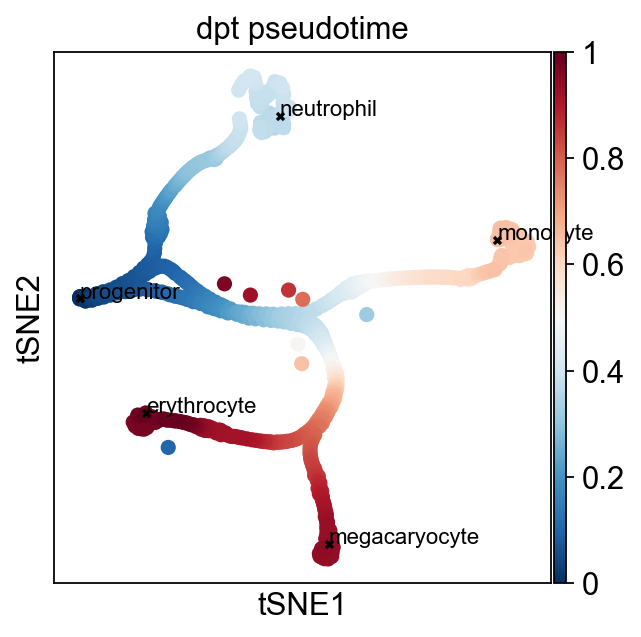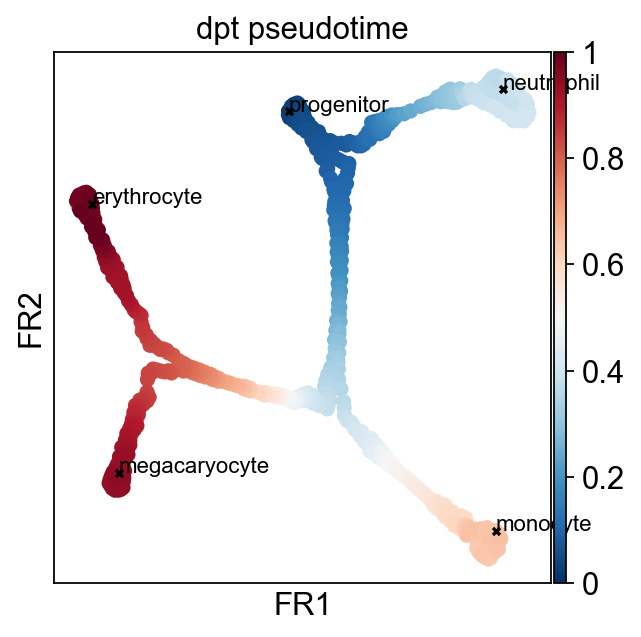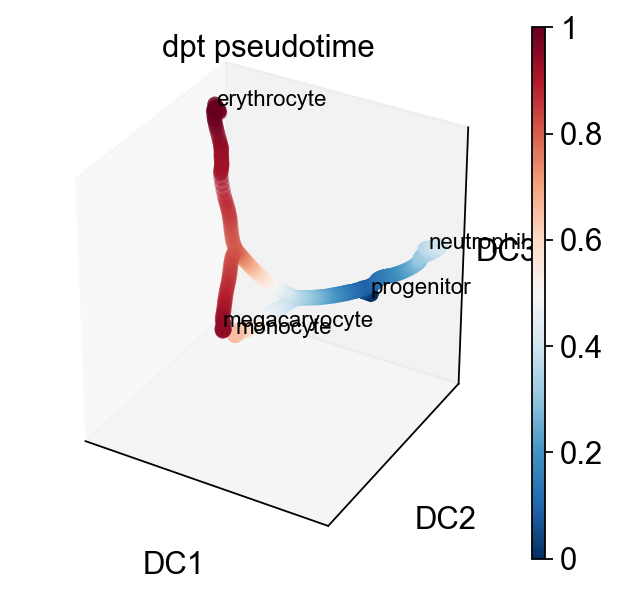The "cuts" into branches are quite arbitrary. Use PAGA instead.

In :
sc.pl.tsne(adata, color='dpt_groups')
sc.pl.draw_graph(adata, color='dpt_groups', title='simulated data: DPT groups')